A Dockerfile that will produce a container with all the dependencies necessary to run this notebook is available here.

In :
%matplotlib inline

In :
import datetime
import logging
from warnings import filterwarnings

In [ ]:
from matplotlib import pyplot as plt
from matplotlib.ticker import StrMethodFormatter
import numpy as np
import pandas as pd
import scipy as sp
import seaborn as sns
from sklearn.preprocessing import LabelEncoder
from theano import pprint

In :
sns.set(color_codes=True)
pct_formatter = StrMethodFormatter('{x:.1%}')

In :
# configure pyplot for readability when rendered as a slideshow and projected
FIG_WIDTH, FIG_HEIGHT = 8, 6
plt.rc('figure', figsize=(FIG_WIDTH, FIG_HEIGHT))

LABELSIZE = 14
plt.rc('axes', labelsize=LABELSIZE)
plt.rc('axes', titlesize=LABELSIZE)
plt.rc('figure', titlesize=LABELSIZE)
plt.rc('legend', fontsize=LABELSIZE)
plt.rc('xtick', labelsize=LABELSIZE)
plt.rc('ytick', labelsize=LABELSIZE)

In :
filterwarnings('ignore', 'findfont')
filterwarnings('ignore', "Conversion of the second argument of issubdtype")
filterwarnings('ignore', "Set changed size during iteration")

# keep theano from complaining about compile locks for small models
(logging.getLogger('theano.gof.compilelock')
.setLevel(logging.CRITICAL))

In :
SEED = 54902 # from random.org, for reproducibility

np.random.seed(SEED)


# The HMC Revolution is Open Source¶

## Probabilistic Programming with PyMC3¶## Who am I?¶### [email protected] • [email protected]¶• Founded 2008, web optimization and personalization SaaS
• Observed 5B impressions and \$4.1B in revenue during Cyber Week 2017monetate.com/about/careers

## Modern Bayesian Inference¶### MCMC Revolution (Diaconis)¶

The Markov Chain Monte Carlo Revolution by famous probabilist Persi Diaconis gives an excellent overview of applications of simulation to many quantitative problems.

### Motivating Examples¶

#### 2017 UK General Election¶YouGov, a polling and opinion data company, correctly called a hung parilment as a result of the 2017 UK general elections in the UK using a Bayesian opinion modeling technique knowns as multilevel regression with poststratification (MRP) to produce accurate estimates of voter preferences in the UK's 650 parliamentary constituences.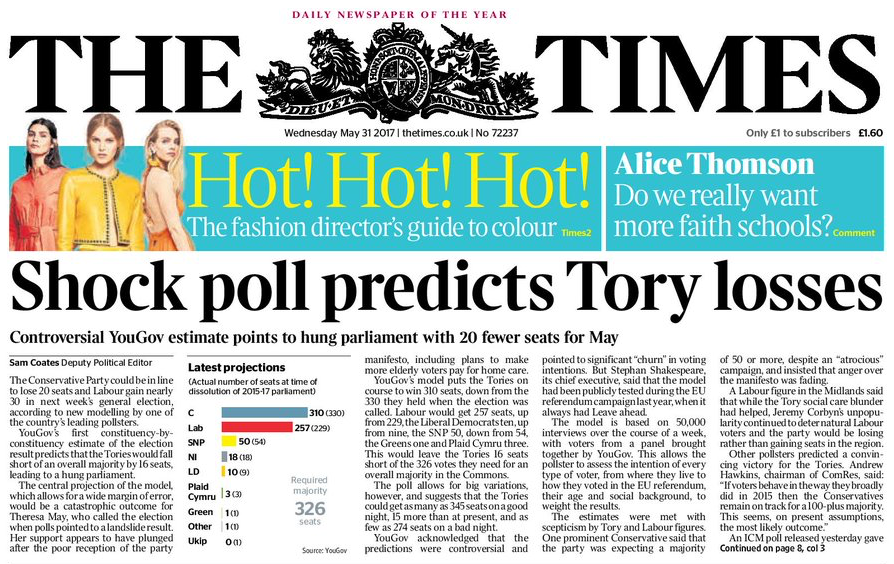Source

#### NBA Foul Calls¶Source

I have done some work using Bayesian methods to study whether or not committing/drawing fouls is a measurable skill among NBA players.

##### Player skills(?)¶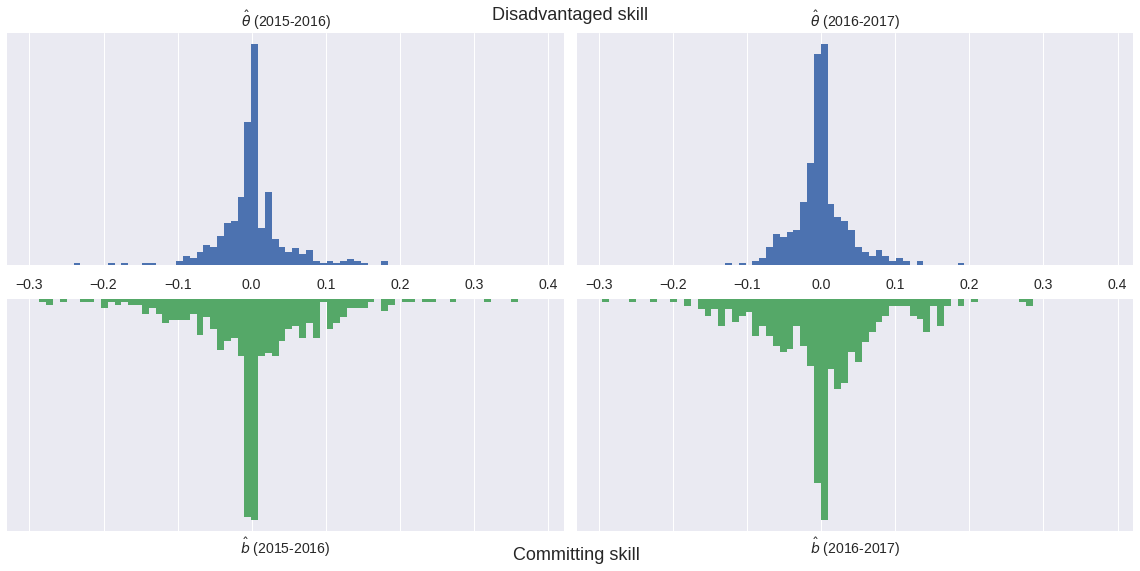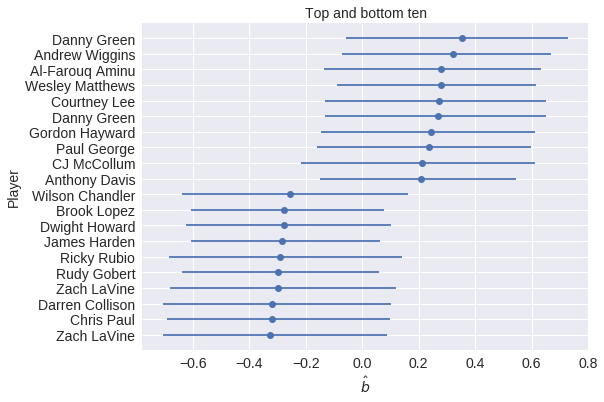## Probabilistic Programming¶

### Data Science — inference enables storytelling### Probabilistic Programming — storytelling enables inference### The Monty Hall Problem¶Initially, we have no information about which door the prize is behind.

In :
import pymc3 as pm

with pm.Model() as monty_model:
prize = pm.DiscreteUniform('prize', 0, 2)


If we choose door one:

 Monty can open Prize behind Door 1 Door 2 Door 3 Door 1 No Yes Yes Door 2 No No Yes Door 2 No Yes No
In :
from theano import tensor as tt

with monty_model:
p_open = pm.Deterministic(
'p_open',
tt.switch(tt.eq(prize, 0),
np.array([0., 0.5, 0.5]), # it is behind the first door
tt.switch(tt.eq(prize, 1),
np.array([0., 0., 1.]),   # it is behind the second door
np.array([0., 1., 0.])))  # it is behind the third door
)


Monty opened the third door, revealing a goat.In :
with monty_model:
opened = pm.Categorical('opened', p_open, observed=2)


Should we switch our choice of door?

In :
CHAINS = 3

SAMPLE_KWARGS = {
'chains': CHAINS,
'random_seed': list(SEED + np.arange(CHAINS))
}

In :
MONTY_SAMPLE_KWARGS = {
'init': None,
'compute_convergence_checks': False,
**SAMPLE_KWARGS
}

In :
with monty_model:
monty_trace = pm.sample(1000, **MONTY_SAMPLE_KWARGS)

monty_df = pm.trace_to_dataframe(monty_trace)

Multiprocess sampling (3 chains in 2 jobs)
Metropolis: [prize]
Sampling 3 chains: 100%|██████████| 4500/4500 [00:02<00:00, 1683.19draws/s]

In :
monty_df['prize'].head()

Out:
0    0
1    0
2    0
3    0
4    1
Name: prize, dtype: int64
In :
ax = (monty_df['prize']
.value_counts(normalize=True, ascending=True)
.plot(kind='bar', color='C0'))

ax.set_xlabel("Door");
ax.yaxis.set_major_formatter(pct_formatter);
ax.set_ylabel("Probability of prize");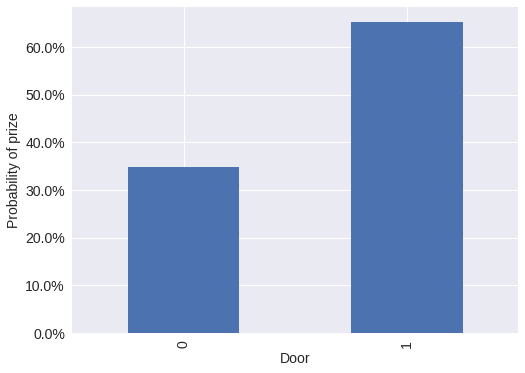#### Probabilistic programming is not new¶BUGS (1989) JAGS (2007)From the PyMC3 documentation:

PyMC3 is a Python package for Bayesian statistical modeling and Probabilistic Machine Learning which focuses on advanced Markov chain Monte Carlo and variational fitting algorithms. Its flexibility and extensibility make it applicable to a large suite of problems.### Monte Carlo Methods¶

In :
N = 5000

x, y = np.random.uniform(0, 1, size=(2, N))

In :
fig, ax = plt.subplots()
ax.set_aspect('equal');

ax.scatter(x, y, c='k', alpha=0.5);

ax.set_xticks([0, 1]);
ax.set_xlim(0, 1.01);

ax.set_yticks([0, 1]);
ax.set_ylim(0, 1.01);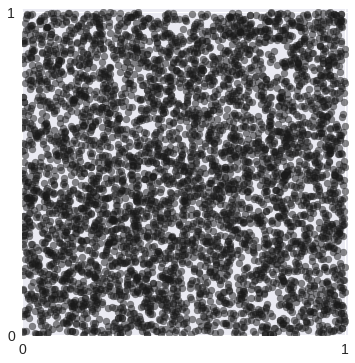In :
fig

Out:In :
in_circle = x**2 + y**2 <= 1

In :
fig, ax = plt.subplots()
ax.set_aspect('equal');

x_plot = np.linspace(0, 1, 100)
ax.plot(x_plot, np.sqrt(1 - x_plot**2), c='k');

ax.scatter(x[in_circle], y[in_circle], c='g', alpha=0.5);
ax.scatter(x[~in_circle], y[~in_circle], c='r', alpha=0.5);

ax.set_xticks([0, 1]);
ax.set_xlim(0, 1.01);

ax.set_yticks([0, 1]);
ax.set_ylim(0, 1.01);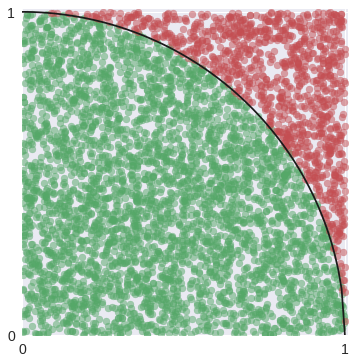In :
fig

Out: